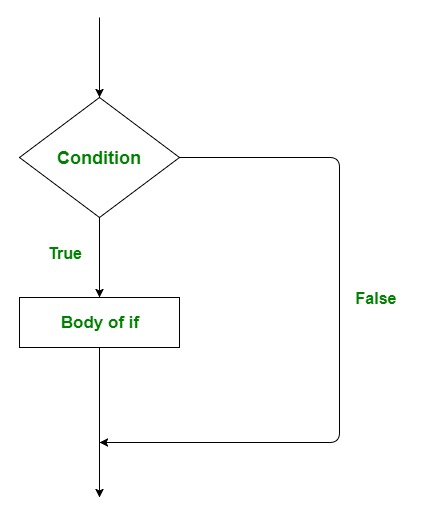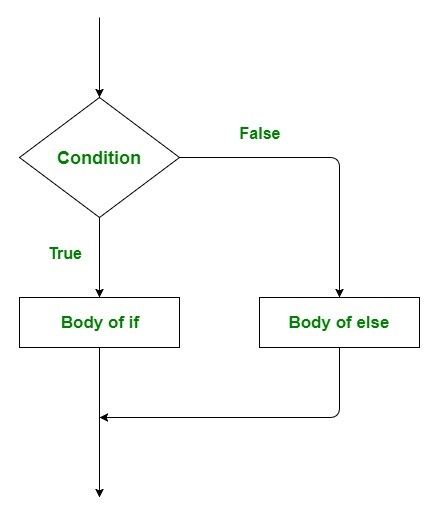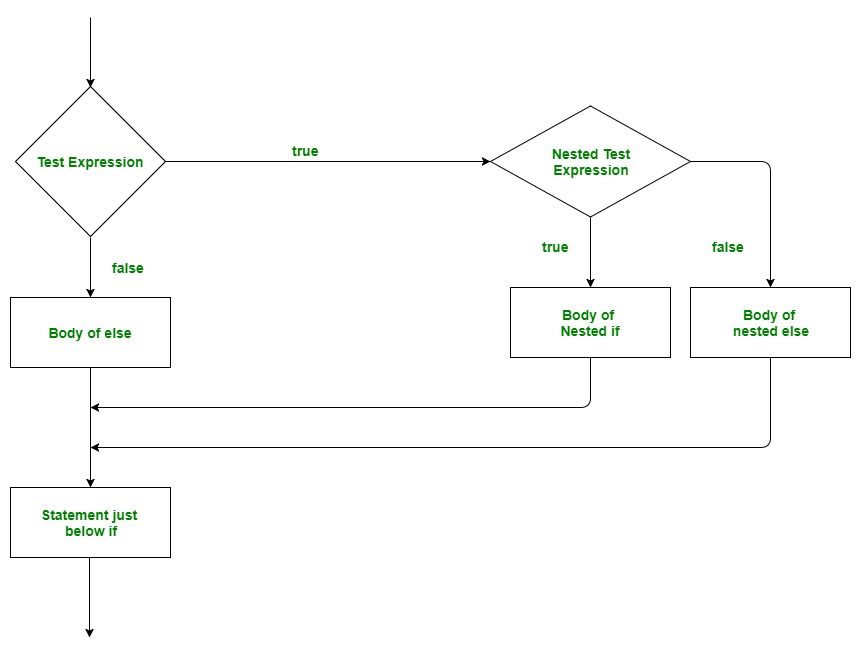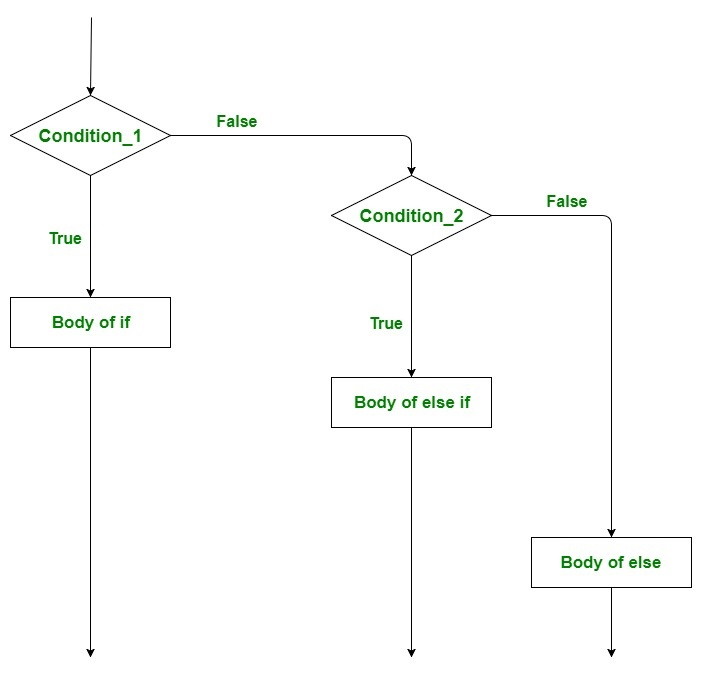# Go Decision Making (if, if-else, Nested-if, if-else-if)

Decision making in programming is similar to decision making in real life. In decision making, a piece of code is executed when the given condition is fulfilled. Sometimes these are also termed as the Control flow statements. Golang uses control statements to control the flow of execution of the program based on certain conditions. These are used to cause the flow of execution to advance and branch based on changes to the state of a program.

The Decision making statements of Go programming are:

### if statement

This is the most simple decision-making statement. It is used to decide whether a certain statement or block of statements will be executed or not i.e if a certain condition is true then a block of statement is executed otherwise not.

Syntax:

```if(condition) {

// Statements to execute if
// condition is true
}
```

Flow Chart:Eample:

 `// Go program to illustrate the  ` `// use of if statement ` `package main ` ` `  `import ``"fmt"` ` `  `func main() { ` `     `  `   ``// taking a local variable ` `   ``var v ``int` `= 700 ` `  `  `   ``// using if statement for  ` `   ``// checking the condition ` `   ``if``(v < 1000) { ` `        `  `      ``// print the following if  ` `      ``// condition evaluates to true ` `      ``fmt.Printf(``"v is less than 1000\n"``) ` `   ``} ` `    `  `   ``fmt.Printf(``"Value of v is : %d\n"``, v) ` `    `  `} `

Output:

```v is less than 1000
value of v is : 700
```

### if…else Statement

The if statement alone tells us that if a condition is true it will execute a block of statements and if the condition is false it won’t. But what if we want to do something else if the condition is false. Here comes the else statement. We can use the else statement with if statement to execute a block of code when the condition is false.

Syntax:

```
if (condition) {

// Executes this block if
// condition is true
} else {

// Executes this block if
// condition is false
}
```

Flow Chart:Example:

 `// Go program to illustrate the  ` `// use of if...else statement ` `package main ` ` `  `import ``"fmt"` ` `  `func main() { ` `     `  `   ``// taking a local variable ` `   ``var v ``int` `= 1200 ` `  `  `   ``// using if statement for  ` `   ``// checking the condition ` `   ``if``(v < 1000) { ` `        `  `      ``// print the following if  ` `      ``// condition evaluates to true ` `      ``fmt.Printf(``"v is less than 1000\n"``) ` `       `  `   ``} ``else` `{ ` `        `  `       ``// print the following if  ` `      ``// condition evaluates to true ` `      ``fmt.Printf(``"v is greater than 1000\n"``) ` `   ``} ` `    `  `} `

Output:

```v is greater than 1000
```

### Nested if Statement

In Go Language, a nested if is an if statement that is the target of another if or else. Nested if statements mean an if statement inside an if statement. Yes, Golang allows us to nest if statements within if statements. i.e, we can place an if statement inside another if statement.

Syntax:

```if (condition1) {

// Executes when condition1 is true

if (condition2) {

// Executes when condition2 is true
}
}
```

Flow Chart:Example:

 `// Go program to illustrate the  ` `// use of nested if statement ` `package main ` `import ``"fmt"` ` `  `func main() { ` `     `  `   ``// taking two local variable ` `   ``var v1 ``int` `= 400 ` `   ``var v2 ``int` `= 700 ` `  `  `   ``// using if statement ` `   ``if``( v1 == 400 ) { ` `        `  `      ``// if condition is true then  ` `      ``// check the following  ` `      ``if``( v2 == 700 )  { ` `           `  `         ``// if condition is true  ` `         ``// then display the following  ` `         ``fmt.Printf(``"Value of v1 is 400 and v2 is 700\n"` `); ` `      ``} ` `   ``} ` `   `  `} `

Output:

```Value of v1 is 400 and v2 is 700
```

Here, a user can decide among multiple options. The if statements are executed from the top down. As soon as one of the conditions controlling the if is true, the statement associated with that if is executed, and the rest of the ladder is bypassed. If none of the conditions is true, then the final else statement will be executed.

Important Points:

• if statement can have zero or one else’s and it must come after any else if’s.
• if statement can have zero to many else if’s and it must come before the else.
• None of the remaining else if’s or else’s will be tested if an else if succeeds,

Syntax:

```if(condition_1) {

// this block will execute
// when condition_1 is true

} else if(condition_2) {

// this block will execute
// when condition2 is true
}
.
.
. else {

// this block will execute when none
// of the condition is true
}
```

Flow Chart:Example:

 `// Go program to illustrate the  ` `// use of if..else..if ladder ` `package main ` `import ``"fmt"` ` `  `func main() { ` `     `  `   ``// taking a local variable ` `   ``var v1 ``int` `= 700 ` `  `  `   ``// checking the condition ` `   ``if``(v1 == 100) { ` `        `  `      ``// if condition is true then ` `      ``// display the following */ ` `      ``fmt.Printf(``"Value of v1 is 100\n"``) ` `       `  `   ``} ``else` `if``(v1 == 200) { ` `        `  `      ``fmt.Printf(``"Value of a is 20\n"``) ` `       `  `   ``} ``else` `if``(v1 == 300) { ` `        `  `      ``fmt.Printf(``"Value of a is 300\n"``) ` `       `  `   ``} ``else` `{ ` `        `  `      ``// if none of the conditions is true  ` `      ``fmt.Printf(``"None of the values is matching\n"``) ` `   ``} ` `} `

Output:

```None of the values is matching
```

My Personal Notes arrow_drop_upCheck out this Author's contributed articles.

If you like GeeksforGeeks and would like to contribute, you can also write an article using contribute.geeksforgeeks.org or mail your article to contribute@geeksforgeeks.org. See your article appearing on the GeeksforGeeks main page and help other Geeks.

Please Improve this article if you find anything incorrect by clicking on the "Improve Article" button below.

Improved By : nidhi_biet

Article Tags :

2

Please write to us at contribute@geeksforgeeks.org to report any issue with the above content.